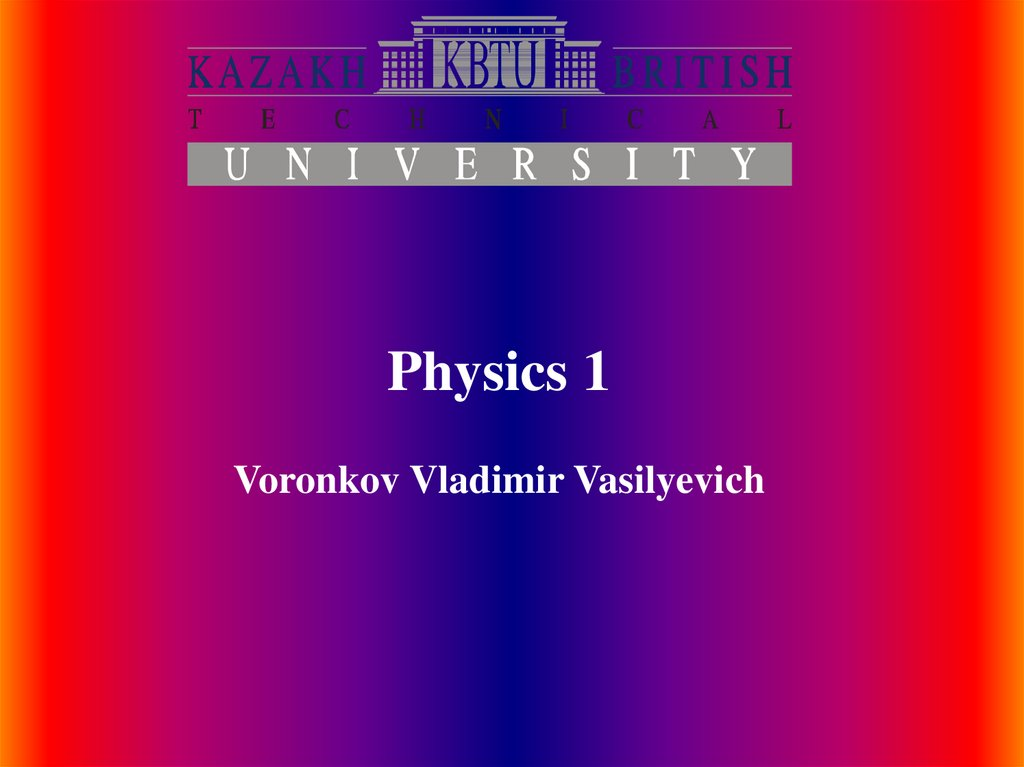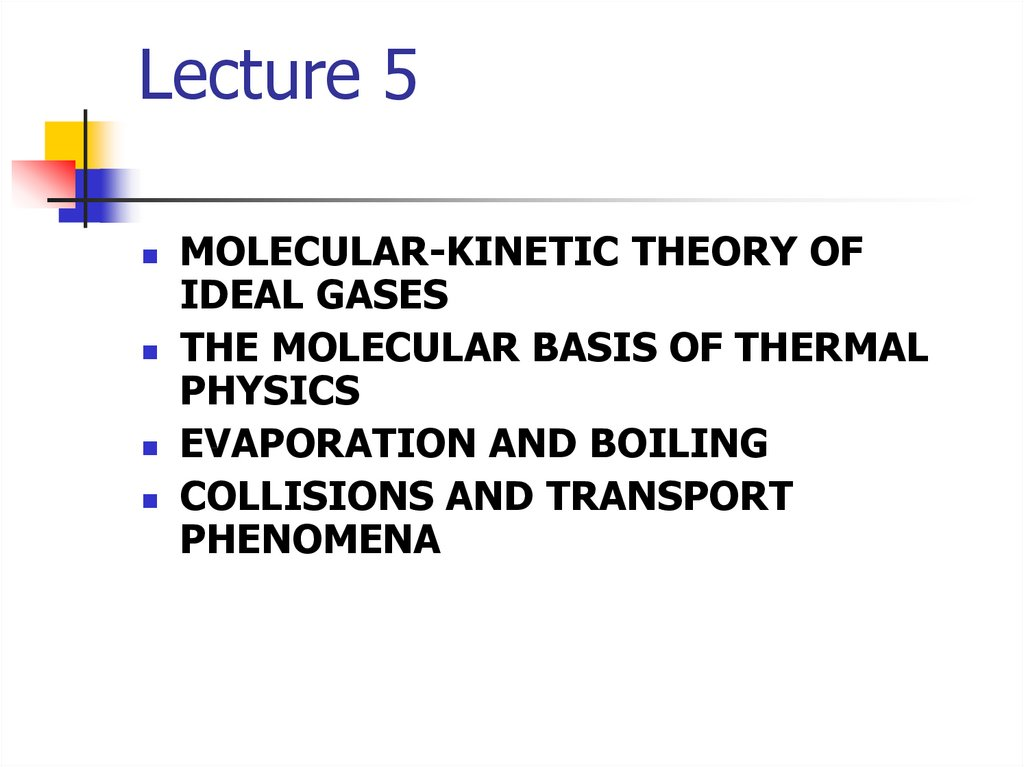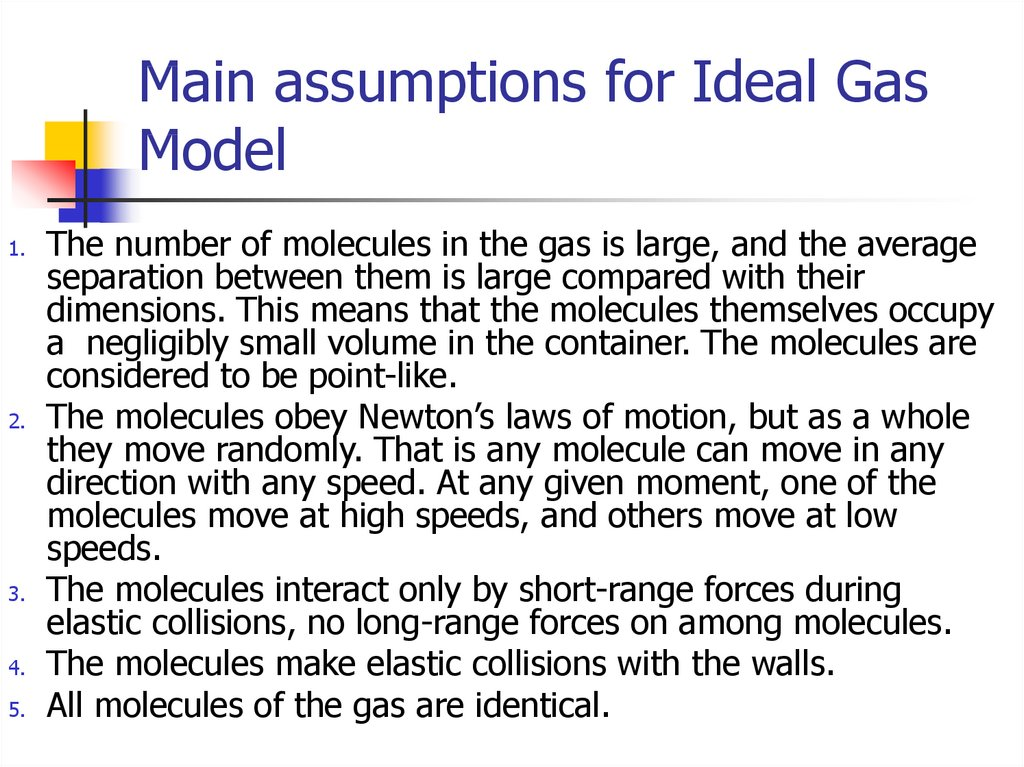# Molecular-kinetic theory of ideal gases. The molecular basis of thermal physics. Lecture 5

Physics 1

## 2. Lecture 5

MOLECULAR-KINETIC THEORY OF
IDEAL GASES
THE MOLECULAR BASIS OF THERMAL
PHYSICS
EVAPORATION AND BOILING
COLLISIONS AND TRANSPORT
PHENOMENA

## 3. Main assumptions for Ideal Gas Model

1.
2.
3.
4.
5.
The number of molecules in the gas is large, and the average
separation between them is large compared with their
dimensions. This means that the molecules themselves occupy
a negligibly small volume in the container. The molecules are
considered to be point-like.
The molecules obey Newton’s laws of motion, but as a whole
they move randomly. That is any molecule can move in any
direction with any speed. At any given moment, one of the
molecules move at high speeds, and others move at low
speeds.
The molecules interact only by short-range forces during
elastic collisions, no long-range forces on among molecules.
The molecules make elastic collisions with the walls.
All molecules of the gas are identical.

## 4. MOLECULAR-KINETIC THEORY OF IDEAL GASES

An ideal gas is in a
cubical container with
sides of length d. We
see i-th molecule, its
velocity Vi, x-component
of velocity is Vxi. Then
is time for travelling
from one side of the
container to another.

## 5.

The change of the i-th molecule
momentum after collision is:
Then the short-term force acting on the
molecule during collision with the wall is:
The long-term force acting on the
molecule in the x-direction is:

## 6.

Using previous expressions we can find xcomponent of the long-term average force
exerted by the wall on the molecule as:
Then by a molecule on the wall:
So the net force of all molecules on the wall:

## 7.

Root mean square (rms) value of x-component of
velocities of N molecules:
Velocity consists of 3 equal components:
So the total force exerted on the wall is:
Then the pressure on the square wall is:

## 8.

N is the number of molecules
This result indicates that the pressure of
a gas is proportional to the number of
molecules per unit volume and to the
average translational kinetic energy of
the molecules

## 9. Molecular interpretation of temperature

So we have
Experimentally found the equation of state for
an ideal gas:
So we can define temperature as:
It tells us that temperature is a direct
measure of average molecular kinetic energy.

## 10. Theorem of equipartition of energy

We can transform the last expression into
Generalization of this result is known as the theorem
of equipartition of energy:
Each degree of freedom contributes 12 k BT to
the energy of a system, where possible
degrees of freedom in addition to translation
arise from rotation and vibration of
molecules.

## 11. Root-mean square speed of molecules

Using the equation of state for an Ideal gas
here n is the number of moles
we can find that

## 12. Internal Energy

In the molecular-kinetic model internal
energy of a gas equals the sum of
kinetic energies of every molecule of
the gas:
N is the number of molecules

## 13. Equation of State for an Ideal Gas

Found experimentally:
n is the number of moles of the gas
R is the universal gas constant:

## 14. The Boltzmann Distribution Law

We found average kinetic energy of a
molecule. But all molecules move
chaotically, collide with each other. So
arises question what is distribution of
molecules depending on their energy.
The answer is given by statistical
mechanics:

## 15. The Boltzmann Distribution Law

Where n0 is defined such that n0dE is the
number of molecules per unit volume having
energy between E = 0 and E = dE.
The Boltzmann law states that the probability
of finding the molecules in a particular energy
state varies exponentially as the negative of
the energy divided by kBT:
n is the number density

## 16. Maxwell–Boltzmann speed distribution function

If N is the total number of molecules,
then the number of molecules with
speeds between V and V+dv is
dN = NV dV.

dN = NV dV.

Root mean square
Mean
Most probable

## 19. Evaporation

We know that liquids evaporate when they’re below
boiling temperature. The speed distribution curve for
molecules in a liquid is described by Maxwell–
Boltzmann function. The molecules that escape the
liquid by evaporation are those that have sufficient
energy to overcome the attractive forces of the
molecules in the liquid phase. Consequently
molecules left behind in the liquid phase have a
lower average kinetic energy; as a result, the
temperature of the liquid decreases. Hence,
evaporation is a cooling process.

## 20.

• .
• In order to evaporate, a mass of water must
collect the large heat of vaporization, so
evaporation is a potent cooling mechanism.
Evaporation heat loss is a major climatic factor
and is crucial in the cooling of the human body.

## 21. Saturation Vapor Pressure

Ordinary evaporation is a surface phenomenon some molecules have enough kinetic energy to
escape. If the container is closed, an equilibrium is
reached where an equal number of molecules return
to the surface. The pressure of this equilibrium is
called the saturation vapor pressure.

## 22.

• The process of evaporation in a
closed container will proceed until
there are as many molecules
returning to the liquid as there are
escaping. At this point the vapor is
said to be saturated, and the
pressure of that vapor is called the
saturated vapor pressure.
• Since the molecular kinetic energy is greater at higher
temperature, more molecules can escape the surface and the
saturated vapor pressure is correspondingly higher. If the liquid
is open to the air, then the vapor pressure is seen as a partial
pressure along with the other constituents of the air. The
temperature at which the vapor pressure is equal to the
atmospheric pressure is called the boiling point.

## 23. Evaporation vs Boiling

• Ordinary evaporation is a surface phenomenon since the vapor pressure is low and since the
pressure inside the liquid is equal to atmospheric
pressure plus the liquid pressure, bubbles of water
vapor cannot form.
• But at the boiling point, the saturated vapor pressure
is equal to atmospheric pressure, bubbles form, and
the vaporization becomes a volume phenomena.

## 24. Boiling Point

• The boiling point is defined
as the temperature at which
the saturated pressure of a
liquid is equal to the
surrounding atmospheric
pressure.
• For water, the vapor pressure
reaches the standard sea
level atmospheric pressure of
760 mmHg at 100°C.
• Since the vapor pressure increases with
temperature, it follows that for pressure greater than
760 mmHg (e.g., in a pressure cooker), the boiling
point is above 100°C and for pressure less than 760
mmHg (e.g., at altitudes above sea level), the boiling
point will be lower than 100°C.

## 25. Collisions

is the collision cross section. Then the
volume it sweeps is
. So this
molecule suffers N=nV collisions (here n is the
number density).

## 26.

Then we can find mean collision time
Considering movement of the target
molecules:
N is the number of molecules
n is the number density

## 27.

Mean free path is an average distance
between collisions:

## 28. Tortuous path of a gas molecule

A randomly moving
molecule has such
displacement:
The displacement of a gas
molecule is proportional to
the square root of the
time.

## 29. Transport Phenomena

By means of collisions that molecules can carry
physical properties (momentum, energy,
concentration, etc.) through a gas. If there is local
heating, that extra thermal energy can be carried to
other parts of the gas through successive collisions.
If some molecules with a particular odour or colour
are introduced in one location, they will spread
through the gas through collisions, even without
convection. The movement of such properties is
called transport phenomena (of thermal energy,
change of concentrations, and so forth), and, more
particularly, the movement of molecules by random
collisions is called diffusion.

## 30. Some terms

The critical temperature of a gas is that temperature
above which the gas will not liquefy, regardless of the amount
of pressure applied to it.
The saturated vapor pressure of a substance is the
additional pressure exerted by vapor molecules on the
substance and its surroundings under a condition of
saturation.
Boiling is defined as vaporization within the body of a liquid
when its vapor pressure equals the pressure in the liquid.
The absolute humidity is defined as the mass of water per
unit volume of air.
The relative humidity can be computed from saturatedvapor-pressure tables according to the following definition:
Relative humidity = actual vapor pressure/ saturated vapor
pressure.

## 31. Van der Waals Gas

n is the number of moles of the gas
Term
represents long-range attraction
between molecules.
Term b - the volume taken up by one mole of
molecules. It accounts for the strong repulsion
between molecules at a characteristic radius.

## 33. NOTE

In this lecture quantity n is used in 2
different ways, each time noting the used
meaning:
n can be the number of moles of the gas
n can be the density number, n=N/V